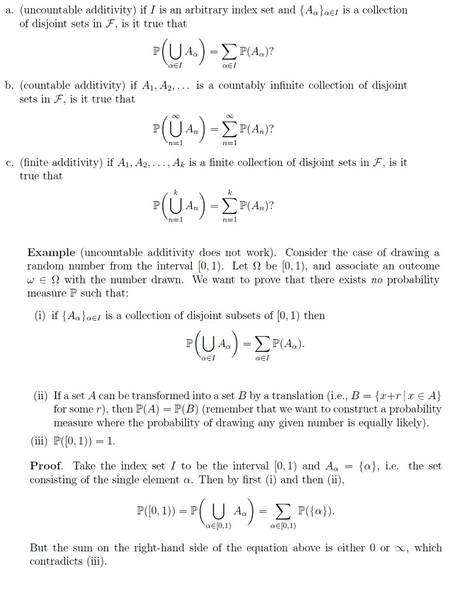# Uncountable additivity (sigma algebra)

woundedtiger4Respected members: I am trying to learn this example but I am stuck that "WHY the sum on the right-hand side of the equation above is either 0 or ∞, which
Can anyone give me an example that when will it 0 and when it will ∞ ?

if I =[0, 1)
lets say that we have three sets A_0 = {0}, A_0.5 = {0.5}. A_1 = {1}
then P(0)=1/3, P(0.5)=1/3, P(1)=1/3
because after all they are just three sets and we are after the probability of A_α
therefore P([0, 1)) = ƩP(A_α) = P(0) + P(0.5) +P(1) = 1/3 + 1/3 + 1/3 = 1 and that satisfies condition that P([0, 1)) = 1

Please correct me if my approach is wrong.

## Answers and Replies

Homework Helper
It follows from (ii). Note that ##A_\alpha = \{ x + r | x \in A_0 \}## with ##r = \alpha## so ##\mathbb P(A_\alpha) = \mathbb P( \{ 0 \} ) \equiv P_0 \in \mathbb R##.
Hence, $$\sum_{\alpha \in [0, 1)} P( A_\alpha ) = |[0, 1)| P_0$$. Now either ##P_0 = 0## in which case the sum will give 0 no matter how many elements you sum over; otherwise ##P_0 > 0## (IIRC a measure must be non-negative) and you sum a positive value over uncountably many elements.

Last edited:
woundedtiger4
It follows from (ii). Note that ##A_\alpha = \{ x + r | x \in A_0 \}## with ##r = \alpha## so ##\mathbb P(A_\alpha) = \mathbb P( \{ 0 \} ) \equiv P_0 \in \mathbb R##.
Hence, $$\sum_{\alpha \in [0, 1)} P( A_\alpha ) = |[0, 1)| P_0$$. Now either ##P_0 = 0## in which case the sum will give 0 no matter how many elements you sum over; otherwise ##P_0 > 0## (IIRC a measure must be non-negative) and you sum a positive value over uncountably many elements.

what is |[0,1)|in ∑α∈[0,1)P(Aα)=|[0,1)|P0 ?

Why the probability of {0} is equal to 0 i.e. P({0}) = P_0 = 0 ? because in (ii) it says "(remember that we want to construct a probability measure where the probability of drawing any given number is equally likely)." does it not say that like other numbers in [0, 1) the 0 has equal probability to appear? or are we considering the number of points in [0, 1) as cantor set (Cantor ternary set) which means that we don't know that how many of them are in [0, 1) ?

PS. I am totally confused because when I look at an example of a fair single coin toss then the probability measure gives 1/2 for head and 1/2 for tail. Similarly, if the probability of drawing any given number is equally likely then why P_0 is zero?

Homework Helper
what is |[0,1)|in ∑α∈[0,1)P(Aα)=|[0,1)|P0 ?
That would be the number of elements in [0, 1), i.e. the number of indices you sum over.
Recall that in the finite case,
$$\sum_{\alpha \in I} P_0 = |A| P_0$$
where |I| is the number of elements in the index set; it may look more familiar as
$$\sum_{k = 0}^n P_0 = n P_0.$$

If you have an infinite sum of a positive constant, the sum will diverge. We write
$$\sum_{k = 0}^\infty P_0 = \infty \qquad \text{( if } P_0 > 0 \text{)}$$
which is just an informal way of saying that
$$\lim_{n \to \infty} \sum_{k = 0}^n P_0 \text{ does not exist}.$$

Why the probability of {0} is equal to 0 i.e. P({0}) = P_0 = 0 ?
It's not necessarily. I said: if it is 0, then the infinite sum is 0. If it is not 0, then the sum is infinite.

•1 person
woundedtiger4
Thanks a tonne. Crystal clearly understood.